##### Actions

(diff) ← Older revision | Latest revision (diff) | Newer revision → (diff)

An integer or fraction associated with two disjoint cycles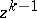and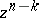in an-dimensional manifoldwhose homology classes are members of the torsion subgroups of the integral homologiesand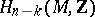, respectively. The simplest example is the linking coefficient of two non-intersecting closed rectifiable curvesin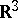, given by the so-called Gauss integral: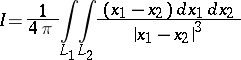(here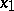andare the radius vectors ofand).

The concept of the linking coefficient generalizes to the case of closed oriented manifolds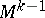and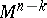in: the linking coefficient is equal to the degree of the mapping (cf. Degree of a mapping)of the oriented direct productinto the sphere, where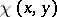,, is the point at whichis cut by a ray through the origin parallel to the vector. The linking coefficient is equal to the intersection index (in homology) of any-chain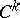such that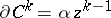with the cycle, divided by. This number is independent of the choice of. If the roles of the cyclesandare interchanged, the linking coefficient is multiplied (in the orientable case) by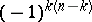. If either of the cycles is replaced by a homological cycle in the complement to the other cycle, the linking coefficient remains the same. This is the basis for the linking interpretation of Alexander duality. If one of the cycles is replaced by a homological cycle, the linking coefficient varies by an integer, thus defining a pairing of torsion subgroups in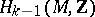andwith values in the quotient group, wheredenotes the set of rational numbers. This pairing establishes a Pontryagin duality between them. In particular, considering the torsion subgroup ofin the case, it defines a non-degenerate quadratic form of self-linkings with values in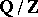, which is a homotopy invariant of the manifold. For example, this was what led to the first observations of asymmetric manifolds, which were particular lens manifolds (cf. Lens space).

Linking coefficients are also considered in other coefficient domains; for example, if a groupis acting freely on the manifold, the homology groups are group modules and the linking coefficient is defined in a suitably localized group ring.

How to Cite This Entry: